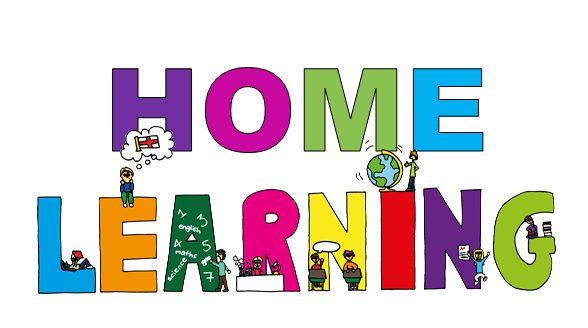# The Beeches Primary School# Year 5 - Summer Home Learning

## English Lessons

1) Setting Description: Reading Comprehension - Fact Retrieval

In this lesson, we are going to learn how retrieve information using a setting description.

2) Setting Description: Reading Comprehension - Fact Retrieval

In this lesson, we are going to learn how retrieve information using a setting description.

3) Setting Description: Identifying the features of a text

In the lesson, we are going to identify the features of a setting description.

4)  Setting Description: SPaG focus - Parenthesis

In this lesson, we are to going to focus on the use of parenthesis in a setting description.

5)  Setting Description: Write a Setting Description

In this lesson, we are going to write a setting description.

## Maths Lessons

1)  To convert between seconds, minutes and hours

In this lesson we are starting a new unit of work on converting measurements. In this lesson we will be looking at time. We will start with a revision of time facts. As the lesson develops, we will hone our skills of converting between seconds, minutes and hours and by the end of this lesson, you will be solving time-related problems on a blank numberline whilst also converting units of time.

2) To solve problems involving converting between hours and minutes

In lesson 2 of our unit on converting measures, we will be deepening our understanding by solving time problems and representing them using bar models. We will work through several problems together which will increase in difficulty. By the end of this lesson, you will have explored the underlying structures which help us convert units of time and solve problems.

3) To convert between units of length

In lesson 3 of our unit on converting measures, we are going to focus on converting between units of length. We will start with a revision of conversion facts between millimetres, centimetres, metres and kilometres. Using these facts, we will apply these to some real life problems around the Olympics. We will also bring in non-standard units thinking about how many laps different track races at the Olympics are.

4)  To apply length conversions to problems

In the fourth lesson of this unit, we will continue to look at length. We will apply everything we have learnt about length so far and apply it to some example of real-life problems. By the end of this lesson, you will be able to convert different units of length so that you can spot errors and solve logistical problems.

5)  To find the perimeter and convert units of measurements

In lesson 5 of our unit on converting measurements, we will be combining our knowledge of perimeter and converting units to find the perimeter of rectilinear shapes.

## Pick and Mix Lessons

Pulse in 3 and 4 time

In this lesson we will be learning about pulse in 3 and 4 time

Who was Siddhartha Gautama?

In this lesson we will be learning about Siddhartha Gautama. He is the first individual to have achieved enlightenment through the practice of meditation. He became known as Buddha, which means ‘the enlightened one’. We will learn about his life and his journey to enlightenment. You will need a piece of paper, a pen and your brain.

El alfabeto y los números

In this lesson we are going to learn about where Spanish is spoken in the world and we are going to learn the Spanish alphabet as well as numbers 1-10. You will need some paper and a pen or pencil for this lesson.